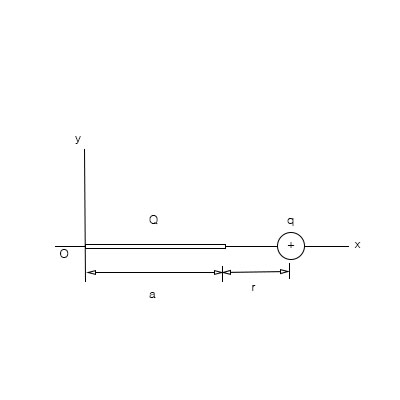# Positive charge Q is distributed uniformly along the x-axis from x=0 to x=a. A positive point...

## Question:

Positive charge {eq}Q {/eq} is distributed uniformly along the {eq}x {/eq} axis from {eq}x=0 {/eq} to {eq}x=a {/eq}. A positive point charge {eq}{/eq} is located on the positive x axis at {eq}x=a+r, {/eq} a distance r to the right of the end of {eq}Q {/eq}.

a) Using integral calculus, calculate the electric field produced by the charge distribution {eq}Q {/eq}at all points {eq}x > a {/eq}.

b) What is the force on the small charge {eq}q {/eq}.

c) If {eq}r>>a {/eq}, what is the force on {eq}q {/eq}?## Electric Field Strength and Electric Force:

Electric field due to a point charge is given by the Coulomb's Law: {eq}E = \dfrac {kQ}{r^2} {/eq}, where k is the Coulomb's constant, Q is the electric charge, and r is the distance to the point of observation. In the case of the system of charges or charge distribution, the electric field is the sum of all fields due to every point charge in the system. The electric force on a charge placed in the electric field is given by {eq}F = Eq {/eq}, where q is the charge magnitude.

a) The electric field is along the positive x-axis and its magnitude can be obtained as follows:

{eq}E = \displaystyle \int \limits_0^a \dfrac {kQ}{a} \cdot \dfrac {dx}{(a+ r - x)^2} {/eq}

Calculating the integral, we obtain:

{eq}E = \dfrac {kQ}{a}\left (\dfrac {1}{r} - \dfrac {1}{a + r} \right ) = \boxed {\color{blue}{\dfrac {kQ}{r (a + r)}}} {/eq}

b) The force on the small charge is:

{eq}F = E q = \boxed {\color{green}{\dfrac {kqQ}{r (a + r)}}} {/eq}

c) In the limit {eq}r \gg a {/eq}, we obtain:

{eq}F \approx \boxed {\color{red}{\dfrac {kqQ}{r^2}}} {/eq}

coinciding with the force between two point charges, as expected,